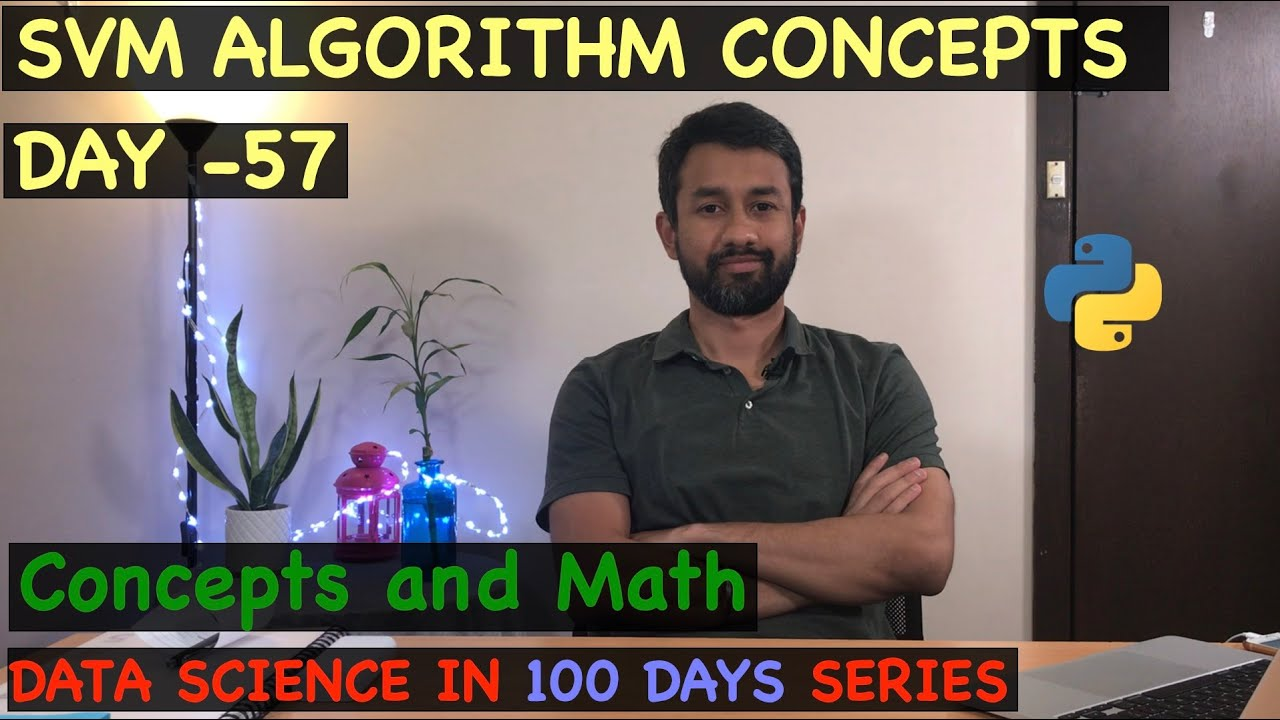1618933178

# SVM Algorithm Concepts - Day 57

This is a video series on learning data science in 100 days. In this video, I have covered the concepts as well as some basic math behind support vector machine algorithm,

• Introduction to SVM
• What type of problems can be solved using Support Vector Machine?
• SVM Terminologies like Support Vector, Margin, Hyperplane, Margin Maximization
• How to generate a hyperplane? What is its mathematical equation?
• What is the linearly separable and linearly non-separable problem? How are they solved?
• Treatment option available for solving a linearly non-separable problem?
• Scenarios, where SVM can be used and not, used

#data-science #developer

## Buddha Community1593396341

## Support Vector Machines(SVM)-What are they?

SVM’s were initially developed in 1960s then they were refined in 1990s and now they are becoming very popular in machine learning as they are demonstrating that they are very powerful and different from other Machine Learning algorithms.

A Support Vector Machine (SVM) is a supervised machine learning algorithm that can be employed for both classification and regression purposes. They are more commonly used in classification problems.

How SVM’s Work?

Consider some usual points on a 2 dimensional space with two columns x1 & x2.

Now how can we derive a line that will separate these two different points and classify them separately? This separation or decision boundary is compulsory as when we add new points in future that we want to classify haven’t been classified yet. We will get to know whether they will fall either in Green area or Red area.

So how to separate these points?

Well there can be numerous ways of drawing lines in between that will achieve the same result as shown.

But we want to find the most optimal line that’s what SVM’s are all about. SVM’ are about finding the best decision boundary that will help us to separate out space into classes.

So lets find out how the SVM’s searches for it. The required line is searched through Maximum Margin.

We can see a line that separates these two classes of points and it has the Maximum Margin which means that the distance between the line and each of these points (touching Red and Green point) is equidistant.

Now sum of these two distances has to be maximized in order for this line to be SVM. The boundary points are know as Support Vectors. Why So?

Basically these two vectors are supporting whole algorithm rest other points don’t contribute to the result of algorithm, only these two points are contributing, therefore they are called Supporting Vectors.

#machine-learning #algorithms #svm #support-vector-machine #artificial-intelligence #algorithms1593347004

## A greedy algorithm is a simple

The Greedy Method is an approach for solving certain types of optimization problems. The greedy algorithm chooses the optimum result at each stage. While this works the majority of the times, there are numerous examples where the greedy approach is not the correct approach. For example, let’s say that you’re taking the greedy algorithm approach to earning money at a certain point in your life. You graduate high school and have two options:

#computer-science #algorithms #developer #programming #greedy-algorithms #algorithms1596427800

## KMP — Pattern Matching Algorithm

Finding a certain piece of text inside a document represents an important feature nowadays. This is widely used in many practical things that we regularly do in our everyday lives, such as searching for something on Google or even plagiarism. In small texts, the algorithm used for pattern matching doesn’t require a certain complexity to behave well. However, big processes like searching the word ‘cake’ in a 300 pages book can take a lot of time if a naive algorithm is used.

# The naive algorithm

Before, talking about KMP, we should analyze the inefficient approach for finding a sequence of characters into a text. This algorithm slides over the text one by one to check for a match. The complexity provided by this solution is O (m * (n — m + 1)), where m is the length of the pattern and n the length of the text.

Find all the occurrences of string pat in string txt (naive algorithm).

``````#include <iostream>
#include <string>
#include <algorithm>
using namespace std;

string pat = "ABA"; // the pattern
string txt = "CABBCABABAB"; // the text in which we are searching

bool checkForPattern(int index, int patLength) {
int i;
// checks if characters from pat are different from those in txt
for(i = 0; i < patLength; i++) {
if(txt[index + i] != pat[i]) {
return false;
}
}
return true;
}

void findPattern() {
int patternLength = pat.size();
int textLength = txt.size();

for(int i = 0; i <= textLength - patternLength; i++) {
// check for every index if there is a match
if(checkForPattern(i,patternLength)) {
cout << "Pattern at index " << i << "\n";
}
}

}

int main()
{
findPattern();
return 0;
}
view raw
main6.cpp hosted with ❤ by GitHub
``````

# KMP approach

This algorithm is based on a degenerating property that uses the fact that our pattern has some sub-patterns appearing more than once. This approach is significantly improving our complexity to linear time. The idea is when we find a mismatch, we already know some of the characters in the next searching window. This way we save time by skip matching the characters that we already know will surely match. To know when to skip, we need to pre-process an auxiliary array prePos in our pattern. prePos will hold integer values that will tell us the count of characters to be jumped. This supporting array can be described as the longest proper prefix that is also a suffix.

#programming #data-science #coding #kmp-algorithm #algorithms #algorithms1624867080

## Algorithm trading backtest and optimization examplesAlgorithm trading backtest and optimization examples.

### xbtusd-vanila-market-making-backtest-hedge